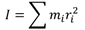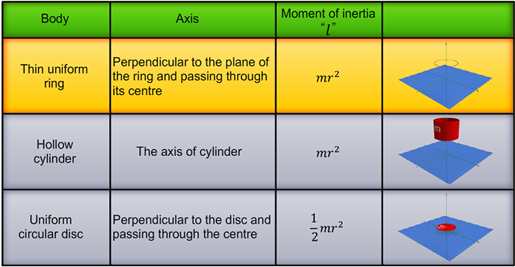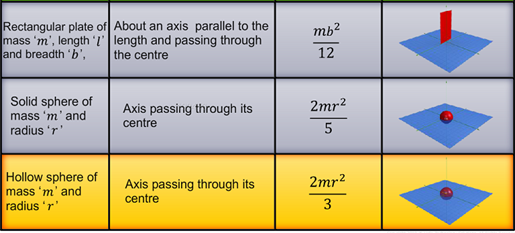Notes On Moment of Inertia of Regular Shaped Bodies - CBSE Class 11 Physics
The moment of inertia of a system of particles is given byFor an isolated body of mass m and at a distance r from the axis of rotation, I = mr2. The moment of inertia of a rigid body depends on its mass and the perpendicular distance of the mass from the axis of rotation. The moment of inertia of the following regular shaped objects can be derived using the methods of integral calculus.#### Summary

The moment of inertia of a system of particles is given byFor an isolated body of mass m and at a distance r from the axis of rotation, I = mr2. The moment of inertia of a rigid body depends on its mass and the perpendicular distance of the mass from the axis of rotation. The moment of inertia of the following regular shaped objects can be derived using the methods of integral calculus.Previous
Next